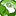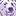# App Development

cancel
Showing results for
Did you mean:Contributor III

## Conditions on a box plot

Hello,

I have a box plot with the following data:

Dimension = Referral Number

Y axis = Reason for treatment

X axis = (Referral commenced date-referral date)

I only want the box plot to display patients aged 0-25 - where do I add age?

I tried the following as my dimension and it didn't work -

Aggr(Only({\$<[Patient Age]={">=0<=25"}>} [Referral Number]), [Referral Number])

Thank you